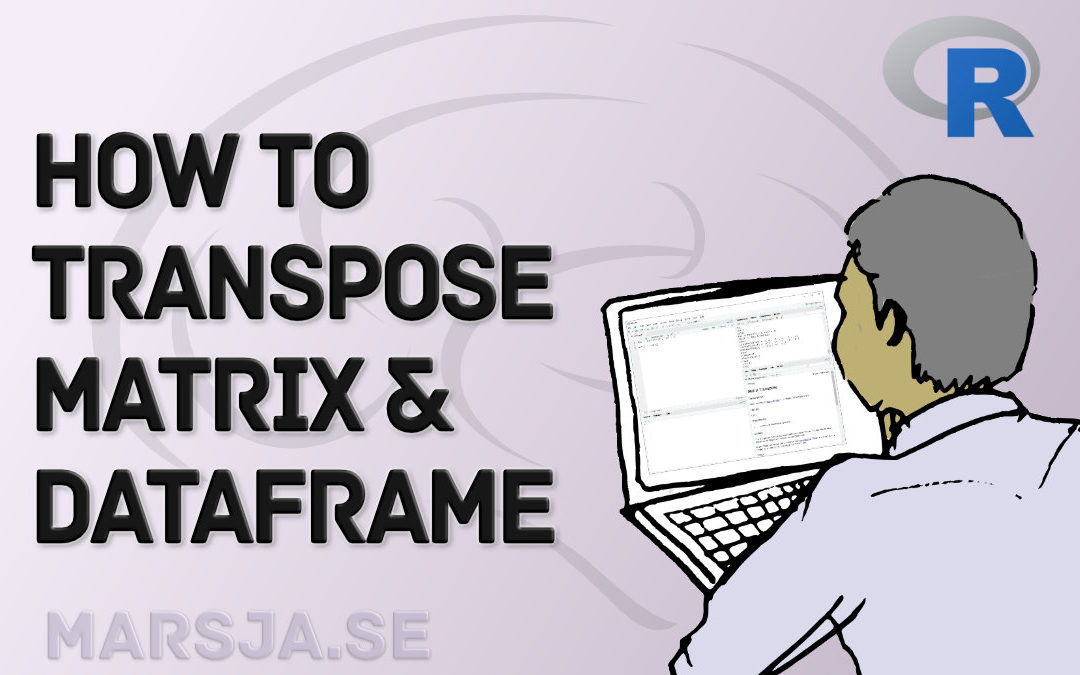## How to Transpose a Dataframe or Matrix in R with the t() Function

In this brief tutorial, you will learn how to transpose a dataframe or a matrix in R statistical programming environment. Transposing rows and columns is a quite simple task if your data is 2-dimensional (e.g., a matrix or a dataframe). If you have a, for example,...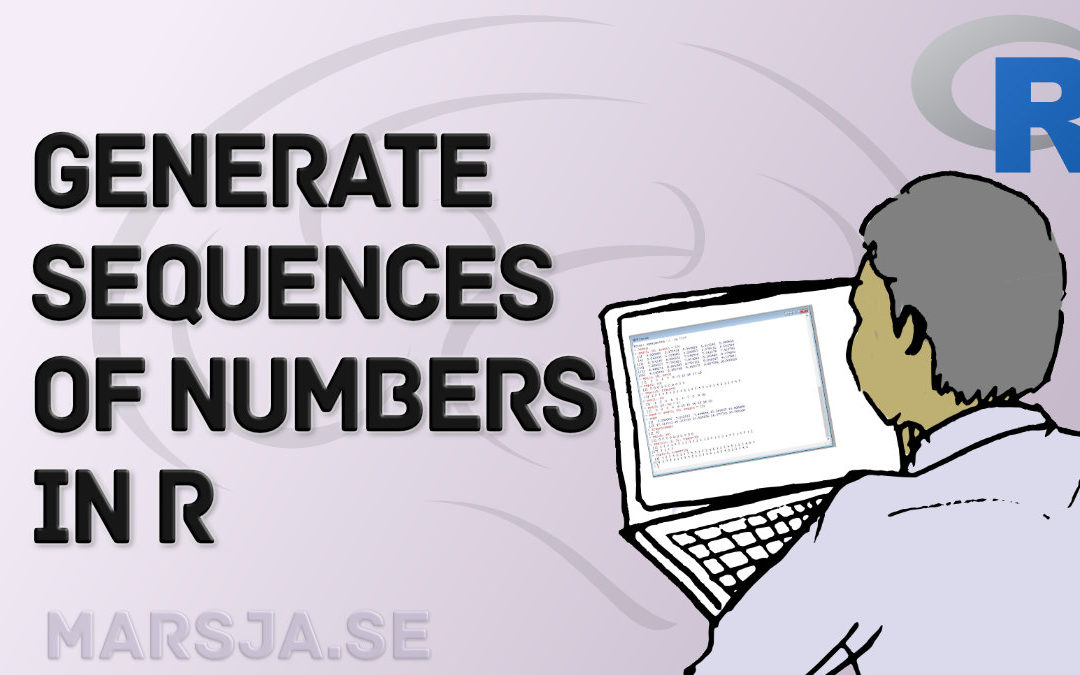## How to Generate a Sequence of Numbers in R with :, seq() and rep()

In this R tutorial, you will learn how to generate sequences of numbers in R. There are many reasons why we would want to generate sequences of numbers. For example, we may want to generate sequences when plotting the axes of figures or simulating data.  Outline...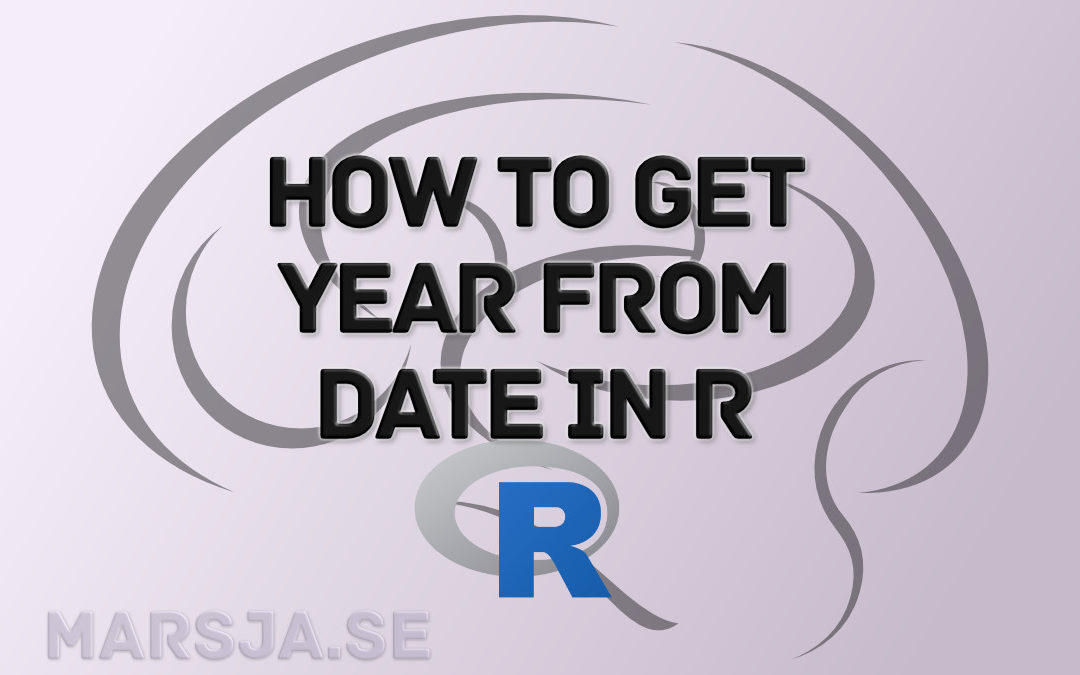## How to Extract Year from Date in R with Examples

In this tutorial, you will learn how to extract year from date in R. You will learn by a couple of examples containing R code along with a description of the code. To know how to extract the year from DateTime may be needed when working with data containing datetime...## How to Add an Empty Column to a Dataframe in R (with tibble)

In this short R tutorial, you will learn how to add an empty column to a dataframe in R. Specifically, you will learn 1) to add an empty column using base R, 2) add an empty column using the add_column function from the package tibble and we are going to use a pipe...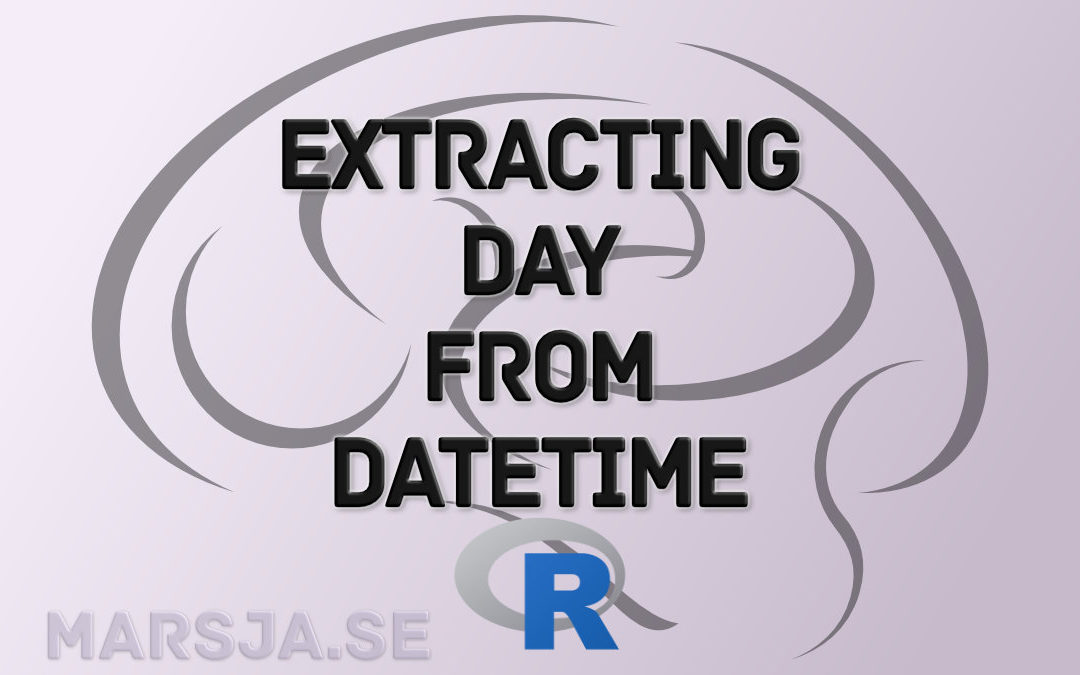## How to Extract Day from Datetime in R with Examples

In this short R tutorial, you will learn how to extract days from datetime in R. This tutorial will contain a couple of examples containing R code and comments about the code. Extracting days from datetime is a task that we may need to do whenever we are working with...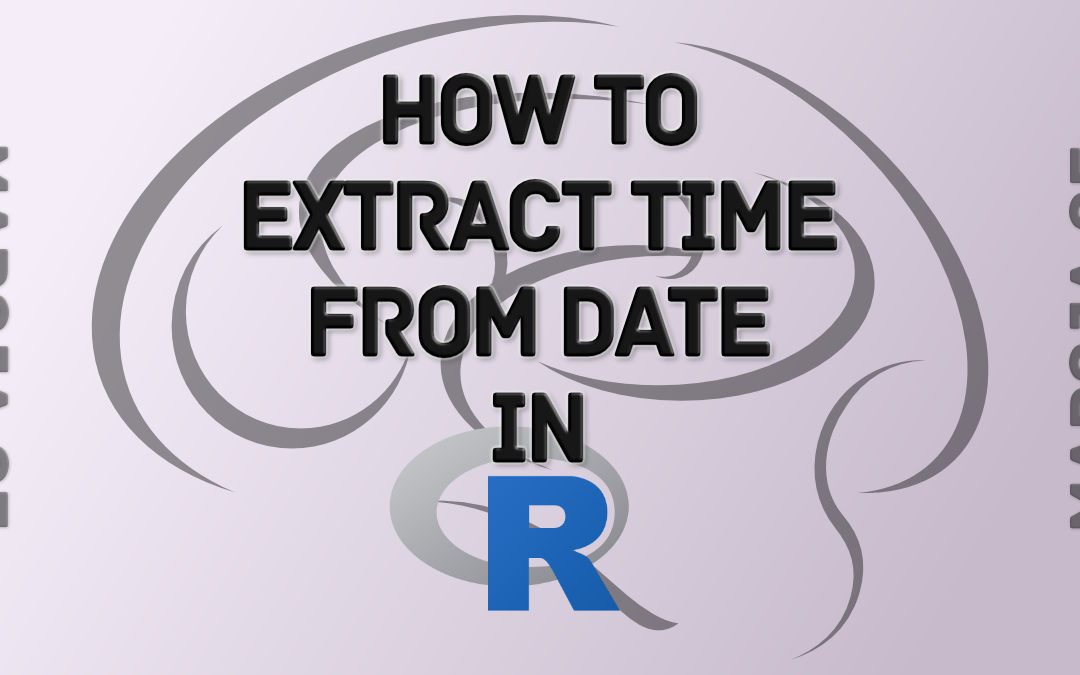## How to Extract Time from Datetime in R – with Examples

In this short R tutorial, you will learn how to extract time from datetime in R by examples. This is a task that may need to be undertaken whenever you have a dataset and you want to do a time series analysis but only want to include time, for example. There are, of...